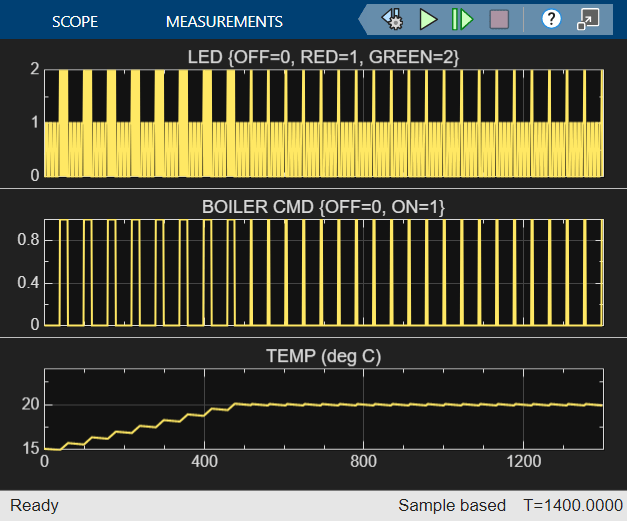Documentation

## Model Bang-Bang Temperature Control System

This example shows how to model a bang-bang control system that regulates the temperature of a boiler. The model has two components:

• Boiler Plant Model is a Simulink® subsystem the models the boiler dynamics.

• Bang-Bang Controller is a Stateflow® chart that implements the bang-bang control logic. The chart uses temporal logic to implement the timing of the bang-bang cycle and 8-bit fixed-point data to represent the temperature of the boiler.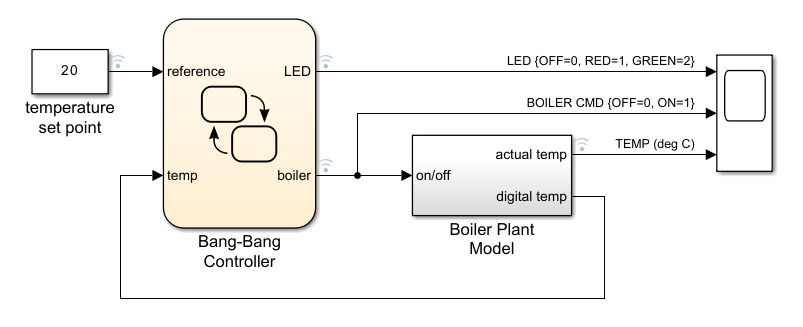### Implement Control Logic by Using `after` Operator

The Bang-Bang Controller determines when the boiler turns on or off. Initially, the boiler is off. After 40 seconds, if the boiler is cold, the boiler turns on. After 20 seconds, the boiler turns off and the bang-bang control cycle repeats.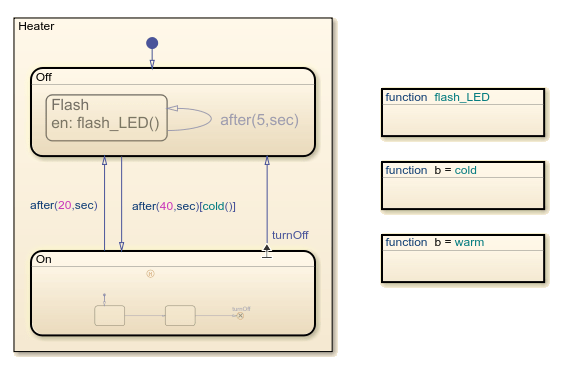To control the transitions between the `On` and `Off` states, the chart calls the absolute-time temporal logic operator `after`. For example, the transition label `after(20,sec)` triggers the transition from `On` to `Off` after the `On` state is active for 20 seconds. The label `after(40,sec)[cold()]` causes the transition from `Off` to `On` to occur if the function `cold` returns `true` after the `Off` state is active for 40 seconds.

The `Off` state also uses temporal logic to control a status LED. Because Stateflow charts in Simulink models do not support the operator `every` for absolute time temporal logic, the state implements the operation of the LED by using a substate `Flash` with a self-loop transition. The transition label `after(5,sec)` triggers the entry action of the substate and causes the LED to flash every 5 seconds.

### Process Floating-Point Data on an 8-Bit Processor

The Boiler Plant Model subsystem simulates the temperature reaction of the boiler during periods of heating or cooling.Depending on the output of the Bang-Bang Controller chart, the subsystem adds or subtracts a temperature increment (+1 for heating or –0.1 for cooling) to the previous boiler temperature and passes the result to the Digital Thermometer subsystem.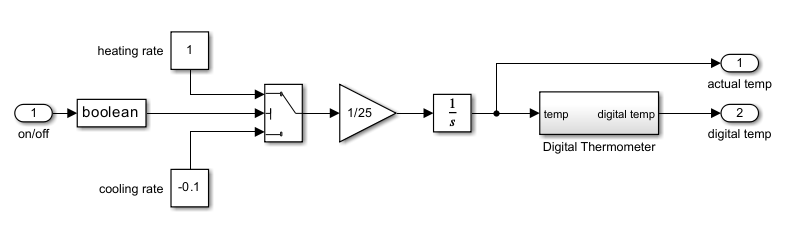The Digital Thermometer subsystem converts the resulting temperature into an 8-bit fixed-point representation. The conversion occurs in three steps.

• The Sensor block converts input boiler temperatureto an intermediate analog voltage output.

• The Analog-to-Digital Converter (ADC) subsystem digitizes the analog voltage from the sensor block by multiplying the voltage by, rounding to the integer floor, and then limiting the result to a maximum of 255 (the largest unsigned 8-bit integer value). The subsystem outputs a quantized integer.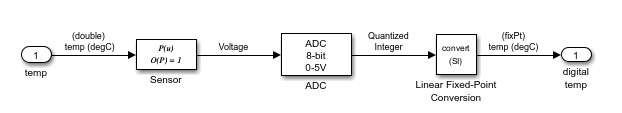• The Linear Fixed-Point Conversion block inverts the combined transfer function of the Sensor and ADC blocks to encode the boiler temperature as a fixed-point number with a slope ofand a bias of. These fixed-point parameters convert the 8-bit quantized integerinto a digital coded temperature.

The Bang-Bang Controller chart receives this digital coded temperature and interprets it as the unsigned 8-bit fixed-point data `temp`. The chart processes this temperature data in an 8-bit environment without any explicit conversions.

### Examine Simulation Results

After simulation, the Simulink scope shows that the boiler reaches a temperature of 20 degrees Celsius after approximately 450 seconds (7.5 minutes). The bang-bang control logic effectively maintains that temperature for the rest of the simulation.